## Other Inverse Trigonometric Functions

To define the inverse tangent, the domain of the tangent must be restricted toThis restricted function is called Tangent (see Figure 1 ). Note the capital “T” in Tangent.Figure 1
Graph of restricted tangent function.

The inverse tangent function (see Figure  2) is defined as the inverse of the restricted Tangent function y = Tan x,Figure 2
Graph of inverse tangent function.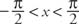Therefore,Identities for the tangent and inverse tangent:The inverse tangent, inverse scant and inverse cosecant functions are derived from the restricted Sine, Cosine, and Tangent functions. The graphs of these functions are shown in Figure  3.Figure 3
Graphs of inverse cotangent, inverse secant, and inverse cosecant functions.

Trigonometric identities involving inverse cotangent, inverse secant, and inverse cosecant: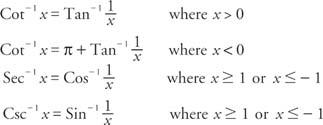Example 1: Determine the exact value of sin [Sec −1 (−4)] without using a calculator or tables of trigonometric functions.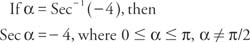In this range, the cosine and the secant are negative in the second quadrant. From this reference triangle, calculate the third side and find the sine (see Figure  4).Figure 4
Drawing for Example 1.Therefore,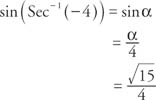Example 2: Determine the exact value of cos (Tan −1 7) without using a calculator or tables of trigonometric functions.In this range, the tangent and the cotangent are positive in the first quadrant. From this reference triangle, calculate the third side and find the cosine (see Figure 5 ).Figure 5
Drawing for Example 2.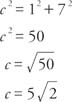Therefore,Graphs of inverse cotangent, inverse secant, and inverse cosecant functions.Graphs of inverse cotangent, inverse secant, and inverse cosecant functions.Graphs of inverse cotangent, inverse secant, and inverse cosecant functions.